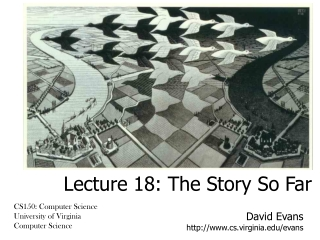DownloadDownload PresentationDavid Evans cs.virginia/evans

# David Evans cs.virginia/evans

Download Presentation## David Evans cs.virginia/evans

- - - - - - - - - - - - - - - - - - - - - - - - - - - E N D - - - - - - - - - - - - - - - - - - - - - - - - - - -
##### Presentation Transcript

1. David Evans http://www.cs.virginia.edu/evans Lecture 18: The Story So Far CS150: Computer Science University of Virginia Computer Science

2. Menu • Finish insert-sort-tree • Course roadmap • Introducing Mutation A few people have extensions on Exam 1, so no talking about the exam questions until Wednesday. If you have an extension on Exam 1, don’t read Chapter 9 until you turn in the exam.

3. insert-one-tree (define (insert-one-tree cf el tree) (if (null? tree) (make-tree null el null) (if (cf el (get-element tree)) (make-tree (insertel-tree cf el (get-left tree)) (get-element tree) (get-right tree)) (make-tree (get-left tree) (get-element tree) (insertel-tree cf el (get-right tree)))))) Each time we call insert-one-tree, the size of the tree approximately halves (if it is well balanced). Each application is constant time. The running time of insert-one-tree is in  (log n)where n is the number of elements in the input tree, which must be well-balanced.

4. insert-sort-helper (define (insert-sort-helper cf lst) (if (null? lst) null (insert-one-tree cf (car lst) (insert-sort-helper cf (cdr lst))))) No change (other than using insert-one-tree)…but evaluates to a tree not a list! (((() 1 ()) 2 ()) 5 (() 8 ()))

5. extract-elements We need to make a list of all the tree elements, from left to right. (define (extract-elements tree) (if (null? tree) null (append (extract-elements (get-left tree)) (cons (get-element tree) (extract-elements (get-right tree))))))

6. Running time of insert-sort-tree (define (insert-one-tree cf el tree) (if (null? tree) (make-tree null el null) (if (cf el (get-element tree)) (make-tree (insert-one-tree cf el (get-left tree)) (get-element tree) (get-right tree)) (make-tree (get-left tree) (get-element tree) (insert-one-tree cf el (get-right tree)))))) n = number of elements in tree (log n) (define (insert-sort-tree cf lst) (define (insert-sort-helper cf lst) (if (null? lst) null (insert-one-tree cf (car lst) (insert-sort-helper cf (cdr lst))))) (extract-elements (insert-sort-helper cf lst))) n = number of elements in lst (n log n)

7. Growth of time to sort random list n2 insert-sort n log2n insert-sort-tree

8. What if tree is not well-balanced? 2 insert-one worst case requires n recursive applications, so insert-sort-tree worst case is in (n2) 3 5 A pathologically unbalanced tree is as bad as a list! 8 13

9. Comparing sorts > (testgrowth best-first-sort) n = 250, time = 110 n = 500, time = 371 n = 1000, time = 2363 n = 2000, time = 8162 n = 4000, time = 31757 (3.37 6.37 3.45 3.89) > (testgrowth insert-sort) n = 250, time = 40 n = 500, time = 180 n = 1000, time = 571 n = 2000, time = 2644 n = 4000, time = 11537 (4.5 3.17 4.63 4.36) > (testgrowth insert-sort-halves) n = 250, time = 251 n = 500, time = 1262 n = 1000, time = 4025 n = 2000, time = 16454 n = 4000, time = 66137 (5.03 3.19 4.09 4.02) > (testgrowth insert-sort-tree) n = 250, time = 30 n = 500, time = 250 n = 1000, time = 150 n = 2000, time = 301 n = 4000, time = 1001 (8.3 0.6 2.0 3.3)

10. Can we do better? • Making all those trees is a lot of work • Can we divide the problem in two halves, without making trees? This is the famous “Quicksort” algorithm invented by Sir Tony Hoare. See Chapter 8. There are lots of ways to do a little bit better, but no way to do asymptotically better. All possible sort procedure have running times in(n log n). (We’ll explain why later in the course...)

11. Synthesis Course Roadmap PS8, 9: Building Web Applications PS7, Ch 13: Meta-Language PS6, Ch 10: Objects Ch 3: Programming PS5, Ch 9: State Ch 4: Procedures Ch 2: Language Ch 5: Data Ch 8: Sorting and Sequencing You are here Ch 13: Tractability Ch 12: Computability Ch 11: Models Ch 7: Time Ch 6: Cost Analysis

12. Computer Science: CS150 so far • How to describe information processes by defining procedures • Programming with procedures, lists, recursion • Chapters 3, 4, 5 • How to predict properties about information processes • Predicting running time, , Ο, Ω • How to elegantly and efficiently implement information processes • Chapter 3 (rules of evaluation)

13. CS150 upcoming • How to describe information processes by defining procedures • Programming with state, objects, networks • How to predict properties about information processes • What is the fastest process that can solve a given problem? • Are there problems which can’t be solved by algorithms? • How to elegantly and efficiently implement information processes • How to implement a Scheme interpreter

14. From Lecture 1: The Liberal Arts language numbers Quadrivium (4 roads) Trivium (3 roads) Grammar Rhetoric Logic Arithmetic Music Geometry Astronomy

15. Liberal Arts Checkup BNF replacement rules for describing languages, rules of evaluation for meaning • Grammar: study of meaning in written expression • Rhetoric: comprehension of verbal and written discourse • Logic: argumentative discourse for discovering truth • Arithmetic: understanding numbers • Geometry: quantification of space • Music: number in time • Astronomy Not much yet… interfaces between components (PS6-9), program and user (PS8-9) Trivium Rules of evaluation, if, recursive definitions Not much yet… wait until April Curves as procedures, fractals (PS3) Quadrivium Yes, listen to “Hey Jude!” Friday: read Neil deGrasse Tyson’s essay